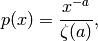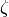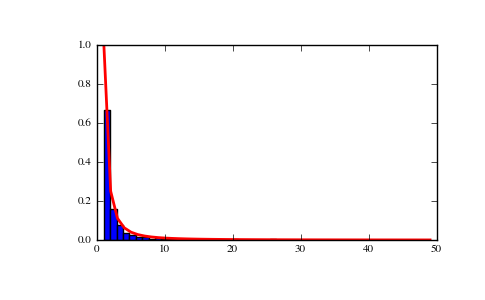# numpy.random.mtrand.RandomState.zipf¶

RandomState.zipf(a, size=None)

Draw samples from a Zipf distribution.

Samples are drawn from a Zipf distribution with specified parameter (a), where a > 1.

The zipf distribution (also known as the zeta distribution) is a continuous probability distribution that satisfies Zipf’s law, where the frequency of an item is inversely proportional to its rank in a frequency table.

Parameters : a : float parameter, > 1. size : {tuple, int} Output shape. If the given shape is, e.g., (m, n, k), then m * n * k samples are drawn. samples : {ndarray, scalar} The returned samples are greater than or equal to one.

scipy.stats.distributions.zipf
probability density function, distribution or cumulative density function, etc.

Notes

The probability density for the Zipf distribution iswhereis the Riemann Zeta function.

Named after the American linguist George Kingsley Zipf, who noted that the frequency of any word in a sample of a language is inversely proportional to its rank in the frequency table.

References

 [R220] Weisstein, Eric W. “Zipf Distribution.” From MathWorld–A Wolfram Web Resource. http://mathworld.wolfram.com/ZipfDistribution.html
 [R221] Wikipedia, “Zeta distribution”, http://en.wikipedia.org/wiki/Zeta_distribution
 [R222] Wikipedia, “Zipf’s Law”, http://en.wikipedia.org/wiki/Zipf%27s_law
 [R223] Zipf, George Kingsley (1932): Selected Studies of the Principle of Relative Frequency in Language. Cambridge (Mass.).

Examples

Draw samples from the distribution:

>>> a = 2. # parameter
>>> s = np.random.zipf(a, 1000)


Display the histogram of the samples, along with the probability density function:

>>> import matplotlib.pyplot as plt
>>> import scipy.special as sps
Truncate s values at 50 so plot is interesting
>>> count, bins, ignored = plt.hist(s[s<50], 50, normed=True)
>>> x = np.arange(1., 50.)
>>> y = x**(-a)/sps.zetac(a)
>>> plt.plot(x, y/max(y), linewidth=2, color='r')
>>> plt.show()